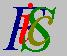Mauro Murzi's pages on Philosophy of Science - Quantum mechanics
Mean values

# [5. Heisenberg indeterminacy principle.]

## Mean values.

The mean value (also known as the expectation or the expected value) E(u) of a physical quantity u can be calculated from the function Ψ using the following formula, where uop is the quantum operator associated to u: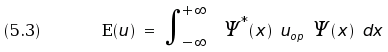In equation (5.3) the operator uop acts on function Ψ . We can also calculate the mean value of u² using the formula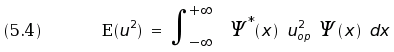The indetermination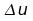of u is given by the equation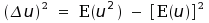. A theorem, due to Paul Ehrenfest, states that the mean values of position and momentum of a particle with mass m satisfy the equations of classical mechanics: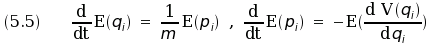Equation (5.5) gives a connection between quantum mechanics and classical mechanics. If the indetermination of position and momentum of a particle is so small that it can be ignored, then the particle acts according to the classical laws of motion.

The amount of the indetermination is given by Heisenberg indeterminacy principle, which affirms that the indeterminationand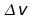of two non commutative entities satisfy the relation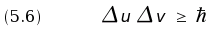If the non commutative entities are position and momentum, the equation (5.6) becomes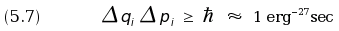Note: 1 erg is the energy of a particle having a mass of 1 gm and a velocity of 1 cm/sec.

Equations (5.7) and (5.5) explain the success of classical mechanics in the realm of macroscopic objects.